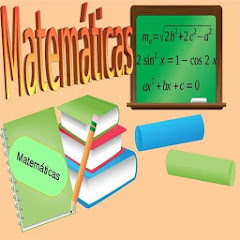# MathPhone Matematica

50K+Everyone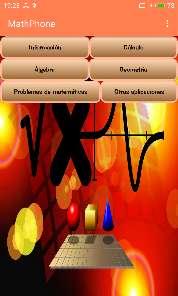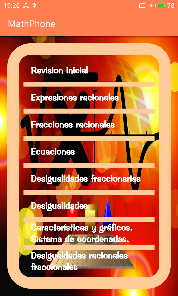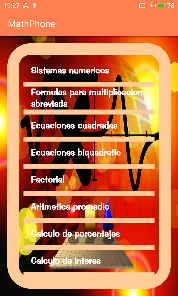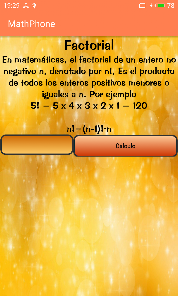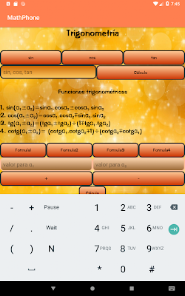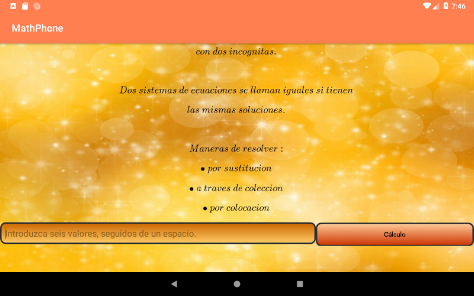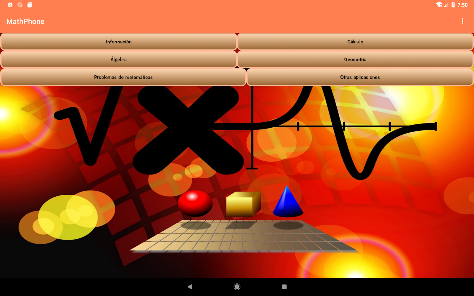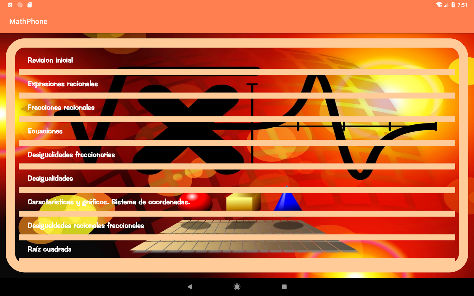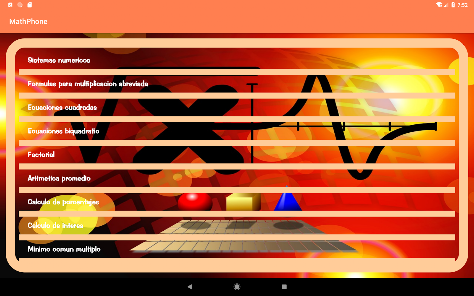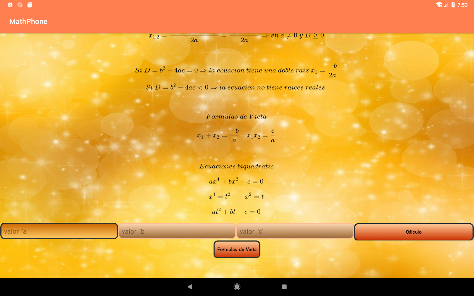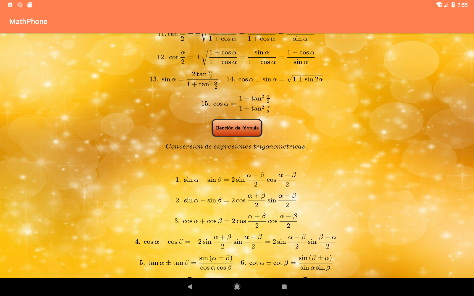Mathematical application with multiple functions. Calculation of percentages, interest rates, equations and much more. Appropriate for students. Each algebra topic contains theory, tasks with a solution where step by step it is shown how they are solved.
At the end of each topic there are tasks that you can try to solve. the app works on both a phone and a tablet.

**** CONTENTS ****

Information

1 .Units of measure
2. Formulas for accidental multiplication
3. Symbols
4. Basic laws
5. Real numbers

Calculation

1. Number systems
2. Formulas for accidental multiplication
3. Square equations
5. Factorial
6. Average arithmetic
7. Calculation of percentages
8. Interest calculation
9. Least common multiple
10. Trigonometry
11. Logarithms
12. Systems of linear equations
13. Arithmetic progression
14. Geometric progression

Algebra

1. Initial review
2. Rational expressions
3. Rational fractions
4. Equations
5. Fractional Inequalities
6. Inequalities
7. Characteristics and graphics. Coordinate system.
8. Fractional Rational Inequalities
9. Square root
10. Square equations
11. Polynomials
12. Irrational expressions
13. Irrational Equations
14. Square function
15. Rational inequalities
16. Root
17. Degree with a real indicator
18. Logarithm. Equations and inequalities
19. Number Rows
20. Arithmetic progression
21. Geometric progression
22. Limit
23. Exponential Function and Logarithmic Functions
24. Trigonometric Equations and Inequalities
25. Continuity of function
26. Derivative of function
27. Function Chart
28. Complex numbers
29. Matrices and determinants
30. Systems of linear equations. Gauss method.
31. Derivatives gives superior order. Differentials.
32. Integrals

Geometry

1. Review
2. Formulas
3. Vectors
4. Triangle and trapezoid
5. Circle
6. Inscribed and described polygons
7. Equivalences
8. Similarity
9. Right Triangle
10. Trigonometric functions at an acute angle
11. Trigonometric functions any angle
12. Scalar product of two vectors
13. Theorem of sines and cosines
14. Formulas for medians and bisectors in a triangle
15. Metric dependencies in a quadrangle
16. Area of ​​a polygon

Maths problems

1. Linear equations and inequalities
2. Equations
3. Degree with an integer pointer
4. Rational expressions
5. Rational equations and inequalities
6. System equations and first degree inequalities
7. Square root
8. Irrational numbers. Square root operations.
9. I triple square. Square equations.
9 II Triple square. Square equations.
10. Inequalities and inequalities of systems of the second and highest degree with an unknown.
11. Irrational equations and inequalities with an unknown
12. Systems of equations of the second degree with two or more unknowns.
13. Arithmetic progression
14. I Logarithmic equations and inequalities
15. II Logarithmic equations and inequalities
16. III Logarithmic equations and inequalities
17. Trigonometry
18. Limit
19. Matrices and determinants
20. Integrals
21. Differential Equations
Updated on
May 20, 2022

## Data safety

Safety starts with understanding how developers collect and share your data. Data privacy and security practices may vary based on your use, region, and age. The developer provided this information and may update it over time.No data shared with third parties